## Correlation Dimension

Define the correlation integral as(1)

whereis the Heaviside Step Function. When the below limit exists, the correlation dimension is then defined as(2)

If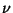is the Correlation Exponent, then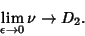(3)

It satisfies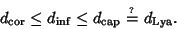(4)

To estimate the correlation dimension of an-dimensional system with accuracyrequires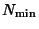data points, where(5)

where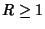is the length of the plateau region.'' If an Attractor exists, then an estimate ofsaturates above somegiven by(6)

which is sometimes known as the fractal Whitney embedding prevalence theorem.